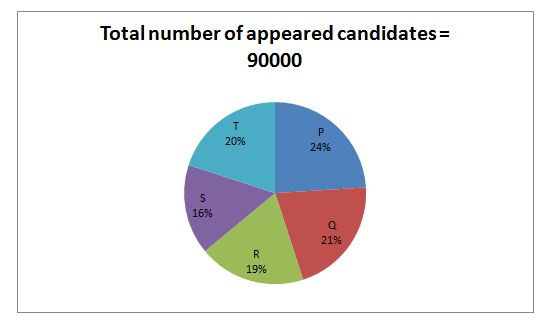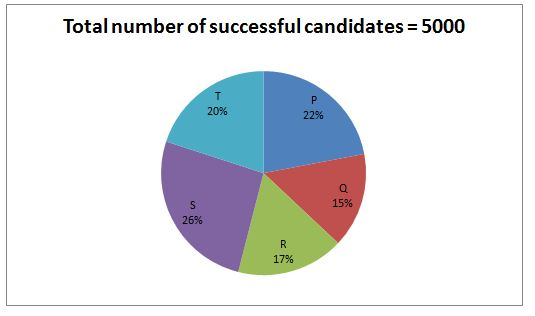# “20-20” Quantitative Aptitude | Crack IBPS RRB PO Prelims 2018 Day-142

Dear Readers, Find the aptitude test questions to crack latest bank exams. We regularly provide 20 aptitude test questions daily for students. Aspirants practice these questions on a regular basis to improve your score in aptitude section. Aspirants preparing for the exams can make use of this “20-20” Quantitative Aptitude Questions. Here we have started New Series of Practice Materials specially for IBPS RRB PO Prelims 2018. Aspirants those who are preparing for the exams can use this “20-20” Quantitative Aptitude Questions.

“20-20” Aptitude Questions | Crack IBPS RRB PO Prelims 2018 Day-142

Click “Start Quiz” to attend these Questions and view Solutions

Directions (Q. 1 – 5): What approximate value should come in the place of question mark (?) in the following questions?

1. ? × 55 = (3564.81 + 775.78) ÷ 35 + 562 + (4/7) of 847 – 58.89
1. 85
2. 74
3. 50
4. 67
5. 98
1. (2/5) of (64/26) of 4145 = 27 % of 2799 + (3/7) of (?)
1. 7900
2. 7200
3. 8600
4. 9400
5. 6500
1. 615 × 1754 ÷ 27 % of 1299 = 45 × 270.19 ÷ 9.21 +? – 4899
1. 6800
2. 6475
3. 6625
4. 5750
5. 5975
1. (14/39) ÷ (167/350) × 216 + 1248.79 – 69 % of 599 = ?
1. 950
2. 1000
3. 800
4. 750
5. 1150
1. (3564 ÷ 12.12) ÷ 3 × 36 + 32% of 4499 – 37 % of 1300.23 = ?
1. 4000
2. 4300
3. 3800
4. 3200
5. 4500

Directions (Q. 6 – 10) In the following questions, two equations I and II are given. You have to solve both the equations and give answer as,

1. If x > y
2. If x ≥ y
3. If x < y
4. If x ≤ y
5. If x = y or the relation cannot be established

6. I. x2 – 17x + 72 = 0

II. 2y2 – 11x + 12 = 0

7. I. x3/4 = 6 × x1/4

II. √y + (1521)1/2 = √2025

8. I. 5x + 9y = 47

II. 3x + 5y = 27

1. I. 5x2 – 12x – 65 = 0

II. 7x2 + 7y – 42 = 0

1. I. 3x2 – 8x – 35 = 0

II. 2y2 + 10y – 28 = 0

1. The present age of Arun is 144 % of the present age of Raghav. The difference between their ages is 22 years. Find the present age of Raghav?
1. 42 years
2. 48 years
3. 50 years
4. 54 years
5. None of these
1. A train whose speed is 35 m/s crosses a bridge in 18 seconds. Another train which is 130 m shorter than the first train crosses the same bridge at the speed of 72 km/hr. Find the time taken by the second train to cross the bridge?
1. 25 sec
2. 32 sec
3. 20 sec
4. 27 sec
5. None of these
1. The amount obtained on Rs. 32000 at an interest 5 % compounded annually for certain period of time is Rs. 37044. Then find the time in years?
1. 2 years
2. 1 year
3. 4 years
4. 3 years
5. None of these
1. The ratio of milk to water in a solution is 3: 1. After adding 15 litres of water to the solution, the ratio of milk to water becomes 6: 7. Then how much milk does the solution contain initially?
1. 21 litres
2. 18 litres
3. 24 litres
4. 15 litres
5. None of these
1. A, B and C started a business by investing Rs. 25000, Rs. 18000 and Rs. 32000 respectively. After 4 months, B invested Rs. 7000 more. And after 3 months, A withdraws 20 % of initial investment. Find the share of C, if the total profit at the end of the year is Rs. 97755?
1. Rs. 40425
2. Rs. 38350
3. Rs. 39275
4. Rs. 41725
5. None of these

Directions (Q. 16 – 20) Study the following information carefully and answer the given questions:

Following pie chart1 shows the total number of students who appeared for IAS exam from 5 different states and the pie chart2 shows the total number of successful candidates of IAS examination from 5 different states.1. Total number of successful candidates of IAS examination from state S is approximately what percentage of total number of candidates appeared for IAS examination from state P?
1. 12 %
2. 25 %
3. 34 %
4. 6 %
5. 18 %
1. Find the ratio between the total number of candidates appeared for IAS examination from state Q and S together to that of total number of successful candidates of IAS examination from state P, Q, R and T together?
1. 333 : 37
2. 111 : 23
3. 75 : 28
4. 52 : 13
5. None of these
1. Find the difference between the total number of unsuccessful candidates of State R to that of State S?
1. 2780
2. 3360
3. 3150
4. 3025
5. None of these
1. Find the average number of unsuccessful candidates of State P, Q and R together?
1. 17500
2. 18300
3. 17900
4. 18700
5. None of these
1. Total number of candidates appeared for IAS examination from state P and Q together is what percentage more/less than the total number of candidates appeared for IAS examination from S and T together?
1. 15 %
2. 20 %
3. 30 %
4. 25 %
5. None of these

Directions (Q. 1 – 5):

55x = (3565 + 776) ÷ 35 + 562 + (4/7) of 847 – 59

55x = (4341/35) + 3136 + (4/7)* 847 – 59

55x = 124 + 3136 + 484 – 59

55x = 3685

X = 67

(2/5) of (65/26) of 4145 = 27 % of 2800 + (3/7) of (?)

4145 = (27/100)*2800 + (3/7)*x

4145 = 756 + (3/7)*x

4145 – 756 = (3/7)*x

3389 = (3/7)*x

3390*(7/3) = x

X = 7908 = 7900

615 × 1754 ÷ [(27/100)*1300] = (45*270)/9 + x – 4900

615 × 1754 ÷ 351 = 1350 + x – 4900

(615*1755)/351 = 1350 + x – 4900

3075 + 4900 – 1350 = x

X = 6625

(14/39) ÷ (168/351) × 216 + 1249 – 69 % of 600 = x

X = (14/39)*(351/168)*216 + 1249 – (69/100)*600

X = 162 + 1249 – 414

X = 997 = 1000

(3564/12) ÷ 3 × 36 + 32% of 4500 – 37 % of 1300 = x

X = (3564/12) *(1/3)*36 + (32/100)*4500 – (37/100)*1300

X = 3564 + 1440 – 481

X = 4523 = 4500

Directions (Q. 6 – 10)

I. x2 – 17x + 72 = 0

(x – 9) (x – 8) = 0

X = 9, 8

II. 2y2 – 11y + 12 = 0

2y2 – 8x – 3y + 12 = 0

2y(y -4) – 3(y – 4) = 0

(2y – 3) (y – 4) = 0

Y = 3/2, 4 = 1.5, 4

X > y

I. x3/4 = 6 × x1/4

X3/4 ÷ x1/4 = 6

X3/4 – ¼ = 6

X2/4 = 6

X1/2 = 6

X = 62 = 36

II. √y + (1521)1/2 = √2025

√y = 45 – 39

√y = 6

Y = 62 = 36

X = y

5x + 9y = 47 –> (1)

3x + 5y = 27 —> (2)

By solving the equation (1) and (2), we get,

X = 4, y = 3

X > y

I. 5x2 – 12x – 65 = 0

5x2 – 25x + 13x – 65 = 0

5x(x – 5) + 13(x – 5) = 0

(5x + 13) (x – 5) = 0

X = -13/5, 5 = -2.6, 5

II. 7y2 + 7y – 42 = 0

7y2 -14y + 21y– 42 = 0

7y(y – 2) + 21 (y – 2) = 0

(7y + 21) (y – 2) = 0

Y = -21/7, 2 = -3, 2

Can’t be determined

I. 3x2 – 8x – 35 = 0

3x2 – 15x + 7x – 35 = 0

3x(x – 5) + 7(x – 5) = 0

(3x + 7) (x – 5) = 0

X = -7/3, 5 = -2.33, 5

II. 2y2 + 10y – 28 = 0

2y2 – 4y + 14y – 28 = 0

2y(y -2) + 14(y – 2) = 0

(2y + 14) (y – 2) = 0

Y = -14/2, 2 = -7, 2

Can’t be determined

The present age of Arun = (144/100)* the present age of Raghav

The ratio of present age of Arun and Raghav = 36: 25 (36x, 25x)

11x = 22

X = 2

Present age of Raghav = 25x = 50 years

Distance covered by the first train= 35*18= 630 m

Distance covered by the second train = 630-130 = 500 m

Time taken by the second train to cross the bridge

= > 500/(72*5/18)

= > (500*18)/(72*5)

= > 25 seconds

Amount = P(1 + r/100)n

37044 = 32000(1 + (5/100))n

(37044/32000) = (105/100)n

(37044/32000) = (21/20)n

(9261/8000) = (21/20)n

(21/20)3 = (21/20)n

n = 3 years

The ratio of milk to water in a solution = 3: 1 (3x, x)

According to the question,

3x/(x + 15) = (6/7)

21x = 6x + 90

15x = 90

X = 6

Initially, Milk = 3x = 18 litres

The share of A, B and C,

= > [25000*7 + 20000*5]: [18000*4 + 25000*8]: [32000*12]

= > 275000: 272000: 384000

= > 275: 272: 384

931’s = 97755

1’s = 105

The share of C = 385’s = Rs. 40425

Directions (Q. 16 – 20)

Total number of successful candidates of IAS examination from state S

= > 5000*(26/100) = 1300

Total number of candidates appeared for IAS examination from state P

= > 90000*(24/100) = 21600

Required % = (1300/21600)*100 = 6 %

The total number of candidates appeared for IAS examination from state Q and S together

= > [(21 + 16)/100]*90000 = 33300

The total number of successful candidates of IAS examination from state P, Q, R and T together

= > [(22 + 15 + 17 + 20)/100]*5000 = 3700

Required ratio = 33300: 3700 = 333: 37

The total number of unsuccessful candidates of State R

= > [90000*(19/100)] – [5000*(17/100)]

= > 17100 – 850 = 16250

The total number of unsuccessful candidates of State S

= > [90000*(16/100)] – [5000*(26/100)]

= > 14400 – 1300 = 13100

Required difference = 16250 – 13100 = 3150

The total number of unsuccessful candidates of State P, Q and R together

= > {[90000*(24/100)] – [5000*(22/100)]} + {[90000*(21/100)] – [5000*(15/100)]} + {[90000*(19/100)] – [5000*(17/100)]}

= > (21600 – 1100) + (18900 – 750) + (17100 – 850)

= > 20500 + 18150 + 16250

= > 54900

Required average = 54900/3 = 18300